## Local maxima detection using SPT

cancel
Showing results for
Did you mean:

## Local maxima detection using SPT

1,059 ViewsNXP Employee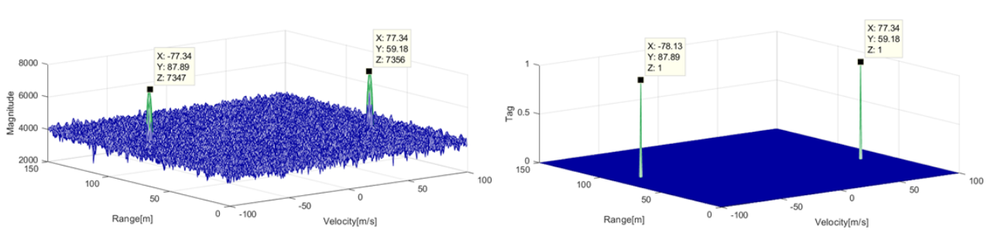# INTRODUCTION

To explain why we need to local maxima detection in RADAR, let us take the simplest scenario. Suppose that we have only one object in front of the RADAR. Then the demodulated signal from a chirp is a sinusoid with the frequency proportional to the range between the RADAR and the object. To find the frequency of the sinusoid we compute its DFT, then we find the peaks of the DFT magnitude. There will be only one peak at frequency of the sinusoid.

# DATA PROCESSING FLOWCHART

Data flow of the MATALB script is presented in the following diagram. The input data for the local maxima detection algorithm is obtained from the SPT input data by applying range FFT, Doppler FFT, and beam forming.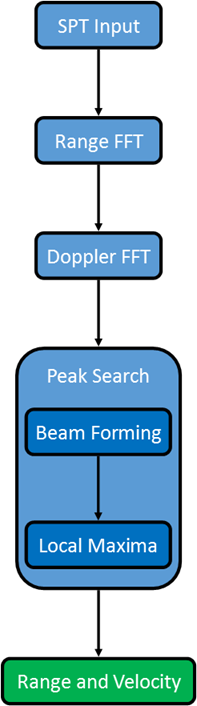Fig. 2: Data processing flowchart

# SPT INPUT DATA

Data received by the SPT is made of demodulated signal and can be organized as a 3 dimensional array in the following way: there is a page for each antenna, and each page contains a matrix where each column contains the samples of a chirp.

NOTE: In MATLAB we refer to the third dimension as pages. For example v(:,:,1) is the first page of v.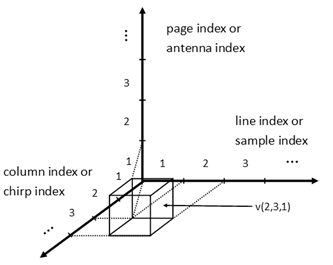Fig. 2: SPT input data representation

% Number of bits used to retain the operands

NBITS = 16;

% Number of bins for histogram

B = 46;

% Maximum range that can be detected

max_range = 150;

% The radar can also detect the negative velocities and its range is in fact [-max_velocity, max_velocity].

max_velocity = 100;

% Number of samples per chirp

M = 512;

% Number of chirps

N = 256;

% Number of antennas

P = 4;

% We take as input the sum of two 3D sinusoids. This is the case when there are two objects in front of the radar.

% The two frequencies in the first dimension or the two frequencies of each chirp

fs1 = 150.5 / M;

fs2 = 100.5 / M;

% The two frequencies in the second dimension or the two Doppler frequencies

fc1 = -99.5 / N;

fc2 =  99.5 / N;

% The two frequencies in the third dimension

fa1 = 1.5 / P;

fa2 = 0.5 / P;

ts = (0:M-1)';

tc = 0:N-1;

ta(1, 1, : ) = 0:P-1;

ts = repmat(ts, [1, N, P]);

tc = repmat(tc, [M, 1, P]);

ta = repmat(ta, [M, N, 1]);

% SPT input data

input_data = sin(2*pi*(fs1*ts + fc1*tc + fa1*ta))+cos(2*pi*(fs2*ts + fc2*tc + fa2*ta))+randn(M, N, P);

input_data = input_data / max(max(max(input_data)));

% SPT input data as 16 bits fixed-point data

input_data_rs = round_and_saturate(input_data, NBITS);

# RANGE FFT

The output array of the range FFT is obtained by replacing each column of the input array with its DFT. As described in the introduction, from each such DFT one can find the ranges of all objects found in front of the RADAR.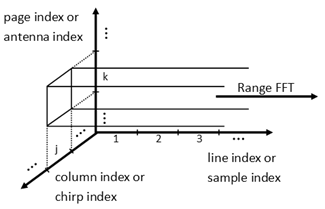Fig. 3: Range FFT input data processing

% Generate window coefficients for range FFT

% In the radar application, the signals of interest are narrowband sinusoids of widely differing strengths (primarily as a consequence of the inverse fourth power variation in radar signal strength with radar range) and the usage of a window function with low sidelobes is crucial for preventing one or more powerful signals from drowning out weaker reflectors.

window_rangeFFT = reshape(window('chebwin', M), [], 1);

window_rangeFFT_rs = round_and_saturate(window_rangeFFT, NBITS);

% Perform Range FFT

% For each line a FFT is performed. The negative frequencies are discarded. The frequencies with significant magnitudes indicates the presents of an object at a specific distance. The distance is proportional with the frequency.

exwindow_rangeFFT = repmat(window_rangeFFT, [1, N, P]);

output_rangeFFT = fft(input_data_rs .* exwindow_rangeFFT, [], 1);

output_rangeFFT = output_rangeFFT(1:end/2, :, : ) / M;

output_rangeFFT_rs = round_and_saturate(output_rangeFFT, NBITS);

# DOPPLER FFT

Doppler FFT has as input the output array of the range FFT. Similar with the range FFT case, the Doppler FFT output array is obtained by replacing each line of the input array with its DFT. From each such DFT one could deduce the velocities of all objects found in front of the radar. More over from the matrix of each page one could deduce the distinct pairs (range, velocities) of all objects found in front of the radar.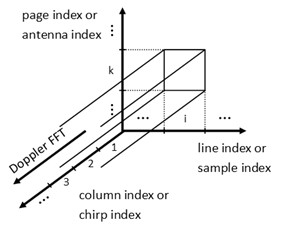Fig. 4: Doppler FFT input data processing

% Generate window coefficients for Doppler FFT

window_dopplerFFT = reshape(window('chebwin', N), [], 1);

window_dopplerFFT_rs = round_and_saturate(window_dopplerFFT, NBITS);

% Perform Doppler FFT

% For each column a FFT is performed. The frequencies with significant magnitudes indicates the presents of an object with a specific velocity. The velocity is proportional with the frequency.

exwindow_dopplerFFT = repmat(reshape(window_dopplerFFT, 1, []), [M/2, 1, P]);

output_dopplerFFT = fft(output_rangeFFT_rs .* exwindow_dopplerFFT, [], 2) / N;

output_dopplerFFT = [output_dopplerFFT(:, end/2 + 1:end, : ) output_dopplerFFT(:, 1:end/2, :)];

output_rangeFFT_rs = round_and_saturate(output_dopplerFFT, NBITS);

# BEAM FORMING

Peak search detects the magnitude peaks of the matrix obtained from beam forming. The beam forming cancels the time delay between the signals of any group of two adjacent antennas and adds the resulted signals. This is accomplished performing an FFT for each page.Fig. 5: Beam forming input data processing

fftOut = fft(output_rangeFFT_rs, 16, 3);

fftOut = abs(fftOut / 16) .^ 2;

% We get data ready to be used by SPT, converting from real data type to log2 data type

fftOut = log2(fftOut) + B;

fftOut_rs = round_and_saturate(fftOut/2^6, 15) * 2^6;

fftMag = squeeze(max(fftOut_rs, [], 3));

% Compute a histogram for each range

rangeHistogram = zeros(M/2, B);

edges = 0:B;

for m = 1:M/2

[tmpHist, ~] = histcounts(fftMag(m, : ), edges);

rangeHistogram(m, : ) = tmpHist;

end

% Determine the threshold for each range

threshold = ones(1, M/2);

for m = 1:M/2

tmpHist = rangeHistogram(m, : );

[~, ind] = max(tmpHist);

while(tmpHist(ind) ~= 0)

ind = ind + 1;

end

threshold(m) = ind-1;

end

# LOCAL MAXIMA DETECTION

Beam forming has as output a matrix. One peak of this matrix represents an object with a specific pair (range, velocity). To find the peaks local maxima detection is used.

The output of the local maxima detection is a matrix that has as line indexes all detectable ranges, and as column indexes all detectable velocities. The matrix contains only 1s and 0s. Each element with value 1 corresponds to least one detected object. The object has its range and velocity that element coordinates.

localMaxInput = fftMag * 2^8;

localMaxThreshold = threshold * 2^8;

outMaxRange = zeros(N, M/2);

outMaxDoppler = zeros(N, M/2);

for m = 1:M/2

% Input Data Type

in_dattyp = 'LOG2';

% Pre-processing

preproc = 'NO_PROCESSING';

% Threshold Compare (valid only for local maxima)

thld_cmp = 'THLD_ENABLED';

% Input Tagged

in_tag = 'NO_TAG';

% Local not Global maxima

loc_n_abs = 'LOCAL_MAX';

% Tag not bitfield (valid only for local maximum calculation)

tag_n_bitfld = 'TAGGED_VEC';

% Cyclic extension (valid only for local maximum calculation)

cyc_extn = 'CYC_EXTN';

% MAXSN enable

maxsn_en = 'MAXSN_DISABLED';

% MAXSN operand Multiplicity select

maxsn_sel = 'MAXS16'; % Don't care

outMaxRange(m, : ) = maxs_mex(complex(localMaxThreshold(m)), complex(localMaxInput(m, :)), in_dattyp, preproc, thld_cmp, in_tag, loc_n_abs, tag_n_bitfld, cyc_extn, maxsn_en, maxsn_sel);

end

for n = 1:N

% Input Data Type

in_dattyp = 'LOG2';

% Pre-processing

preproc = 'NO_PROCESSING';

% Threshold Compare (valid only for local maxima)

thld_cmp = 'THLD_DISABLED';

% Input Tagged

in_tag = 'TAGGED';

% Local not Global maxima

loc_n_abs = 'LOCAL_MAX';

% Tag not bitfield (valid only for local maximum calculation)

tag_n_bitfld = 'PACKED_BITFLD';

% Cyclic extension (valid only for local maximum calculation)

cyc_extn = 'NO_CYC_EXTN';

% MAXSN enable

maxsn_en = 'MAXSN_DISABLED';

% MAXSN operand Multiplicity select

maxsn_sel = 'MAXS16'; % Don't care

outMaxDoppler(:, n) = maxs_mex(complex(0), complex(outMaxRange(:, n)), in_dattyp, preproc, thld_cmp, in_tag, loc_n_abs, tag_n_bitfld, cyc_extn, maxsn_en, maxsn_sel);

end

# RESULT

The fist image below is the beam forming result which is also the local maxima detection input, and the second image below is the local maxima detection output.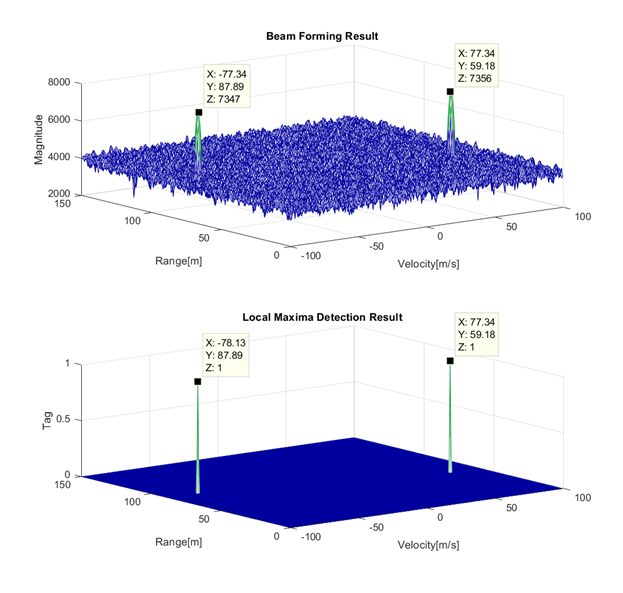Fig. 6: Input and output of Local maxima detection

The script used in this example is attached below.

We hope you find this information useful. Feel free to LIKE this article and comment below.

0 Replies